# Revisal Problems (Past 13 Years) JEE Advanced (States Of Matter)

## 20 Questions MCQ Test Chemistry for JEE Advanced | Revisal Problems (Past 13 Years) JEE Advanced (States Of Matter)

Description
Attempt Revisal Problems (Past 13 Years) JEE Advanced (States Of Matter) | 20 questions in 40 minutes | Mock test for JEE preparation | Free important questions MCQ to study Chemistry for JEE Advanced for JEE Exam | Download free PDF with solutions
QUESTION: 1

Solution:
QUESTION: 2

Solution:
QUESTION: 3

### The pressure of gaseous mixture of CO and CO2 gasesi ncreased from 2.0 kPa to 5.0 kPa on passing through heated charcoai under ideal conditions. Thus mole fraction of CO in the original mixture is

Solution:
QUESTION: 4

Vapour pressure of H2O (g) in sample of air is 35 torr at 320 K. Their molar concentration of water vapour in air is

Solution:
QUESTION: 5

The total pressure of a mixture of H2 and O2 is 1.00 bar. The mixture is allowed to react to form water, which is completely removed to leave only pure H2 at a pressure of 0.35 bar.
Thus, mole fraction of H2 in the original mixture is

Solution:
QUESTION: 6

The following diagram shows the Maxwell speed distribution curves for a certain ideal gas at two different temperatures (T1 and T2).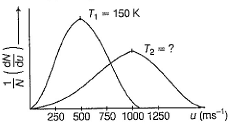Temperature T2 is

Solution:
QUESTION: 7

The volume of 1.00 mole of an ideal gas at 1.00 bar and 0°C decreased to 21.1 L when placed in a methanoi-ice bath. Also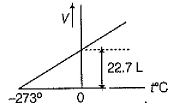Q. Thus, temperature of the methanoi-ice bath is

Solution:
QUESTION: 8

Hot air balloons are being used for experimental study. Assume volume of the balloon is 100 m3, the temperature of air is 25°C, the pressure is 1.00 bar and air is an ideal gas with an average molar mass of 29.0 g mol-1. Temperature of the hot air balloon needed to lift 1,00 kg of mass is

Solution:
QUESTION: 9

Direction (Q. No. 9) This section is based on Statement I and Statement II. Select the correct answer from the codes given below.

Q.

Stement I : Second virial coefficient of CO2 is - 0.065 L mol-1 at 400 K and 30 atm making CO2 more compressible than an ideal gas.

Statement II : Compressibility factor of CO2 (Z = 0.945) is less than 1 (Z = 1 for ideal gas) due to the attractive forces betw een CO2 molecules.

Solution:
*Multiple options can be correct
QUESTION: 10

Direction (Q. Nos. 10 and 11) This section contains 2 multiple choice questions. Each question has four choices (a), (b), (c) and (d), out of which ONE or MORE THAN ONE are correct.

Q. Which of the following statements is/are true when comparing 0.5 mole H2(g) and 1.0 mole He(g)at STP?

The two gases have equal

Solution:
*Multiple options can be correct
QUESTION: 11

At 300 K and 100 atm, the second virial coefficient (B) of N2 and CH4 are - 4.2 cm3 mol-1 and - 15 cm3 mol-1 respectively. Thus

Solution:
QUESTION: 12

Direction (Q. Nos. 12-17) This section contains three paragraphs, each describing theory, experiments, data etc. Six questions related to the paragraphs have been given. Each question has only one correct answer among the four given options (a), (b), (c) and (d).

Passage I

Second virial coefficient (B) of methane is - 0.042 L mol-1 at 300 K and 100 atm.

Q. Compressibility factor the gas under given condition is

Solution:
QUESTION: 13

Passage I

Second virial coefficient (B) of methane is - 0.042 L mol-1 at 300 K and 100 atm.

Q. Difference in molar volume (L mol-1) under ideal and real conditions is

Solution:
QUESTION: 14

Passage Il

A balloon of radius 10 m, mass of 100 kg is filled with helium at 1.66 bar and 27°C. Density of air is 1.2 kg/m3 and R = 0.083 bar dm3 K-1 mol-1.

Q. If balloon goes up, mass of displaced air is

Solution:
QUESTION: 15

Passage Il

A balloon of radius 10 m, mass of 100 kg is filled with helium at 1.66 bar and 27°C. Density of air is 1.2 kg/m3 and R = 0.083 bar dm3 K-1 mol-1.

Q. Pay load {lifting capacity) is

Solution:
QUESTION: 16

Passage III

When attractive forces are negligible, Charles’ law for a non-ideal gas can be represented by following isobar (at 1 bar).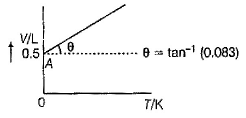Q. Equation representing this isobar is

Solution:
QUESTION: 17

Passage III

When attractive forces are negligible, Charles’ law for a non-ideal gas can be represented by following isobar (at 1 bar).Q. From this graph it is concluded that

Solution:
*Answer can only contain numeric values
QUESTION: 18

Direction (Q. Nos. 18 and 19) This section contains 2 questions. Each question, when worked out will result in an integer from 0 to 9 (both inclusive).

Q. Nickel forms a gaseous compound of the formula Ni(CO)x. What is the value of x given the fact under the same conditions of temperature and pressure, methane (CH4) effuses 3.3 times faster than the compound?

Solution:
*Answer can only contain numeric values
QUESTION: 19

34.05 mL of phosphorus vapour px weighs 0.0625 g at 546°C and 1 bar pressure. What is the value of x?

Solution:
QUESTION: 20

Direction (Q. No. 20) Choices for the correct combination of elements from Column I and Column II are given as options (a), (b), (c) and (d), out of which one is correcf.

Q. 1.00 mole sample of O2 and 3.00 moles sample of H2 are mixed isothermally in a 100.0 L container at 400 K.

Match the partial pressures of different species in Column I with their values, in Column II and select answer from codes given at the end.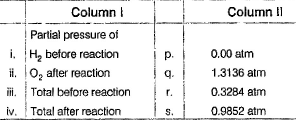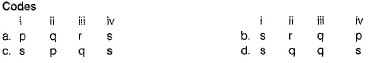Solution:Use Code STAYHOME200 and get INR 200 additional OFF Use Coupon Code